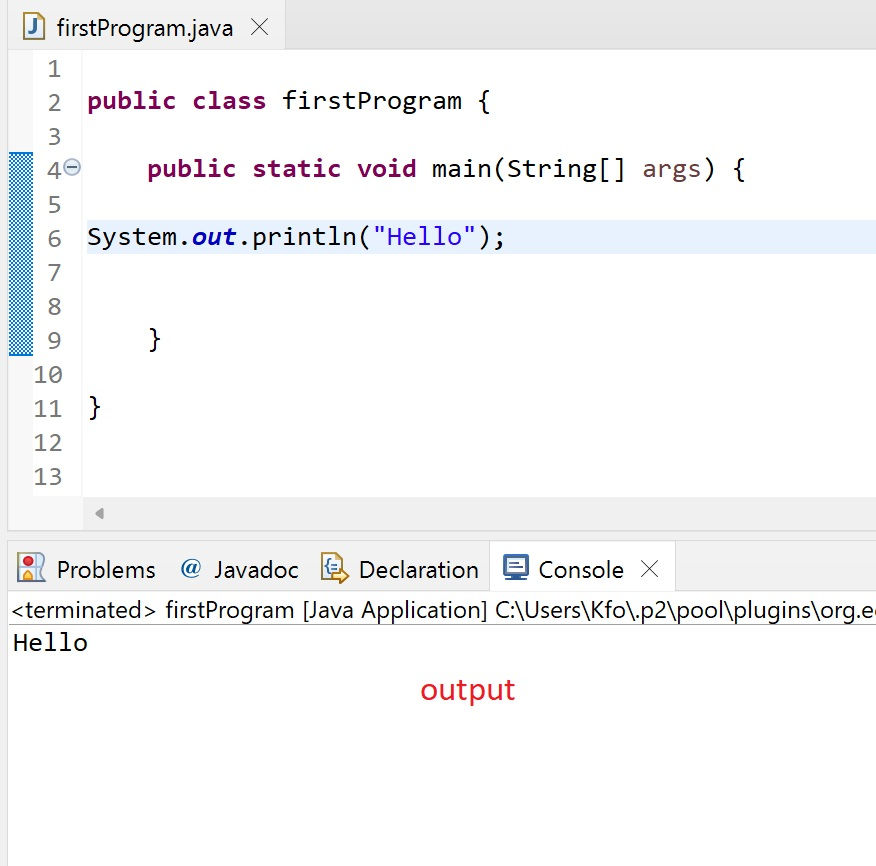•A.razaq

# T5- println and print methods

the print function in java ( method) comes in different types for different purposes

the first one we will learn is the most commonly used which is the " println"

which stands for print line

we use it to print and display a result then the cursor will move to the next line after

`System.out.println();`

if we want to print text and String we must enclose our message between double quotation marks " "

for example if we want to print the word Hello

`System.out.println("Hello");`we learned in the pervious tutorial how to assign and declare variables

so in this example we can see how we can use the println method to call a variable value

```int x = 5;
System.out.println(x);```

we declared an integer variable named x and gave it the value of 5

now when we use the println to call for the variable we dont use the double quotations marks

the result will be like thisif we use the "" double quotations it will only display x as a string not as the variable

the difference between print and println is

print keeps the cursor on the same line , while println moves it to the next line

for example

les say we want to print " x value is " then we display the value of the variable x

```int x =5;
System.out.print(X value = );
System.out.print(x);```

space is included so we make space after = sign

the output will benow lets try to use print and println together and see the difference

```	int x = 5;
System.out.println("x value = ");
System.out.print(x);  ```

the output will be like thisprintln will display its result in a separate line and move to the next line

-----------

we can display multiple lines of texts using one println statment

by using the new line character

`\n `

lets say we want to display a message and we want the output to be in multiple lines , like this for example

```welcome
to my
java
tutorials ```

we can simply use the new line character like this

`System.out.println("Welcome\nto my\njava\ntutorials"); `

we place ( \n) before every part we want to be on a single line

so the output will be---

so its obviously better to use the new line character instead of using multiple println statements

this

`System.out.println("Welcome\nto my\njava\ntutorials"); `

will give the same result as this

```System.out.println("Welcome");
System.out.println("to my");
System.out.println("java");
System.out.println("tutorials");```

the difference is obvious in term of effort and having a better clean code